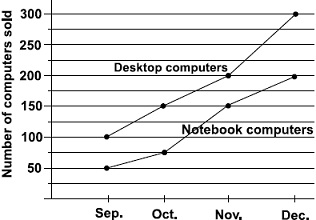# User Forum

Subject :IMO    Class : Class 6

The line graph shows the number of desktop and notebook computers sold at Beena's electronic store from September to December.How many more desktop than notebook computers did the store sell ?

A305
B250
C275
D285

I think that the starting number on y axis should Be 0 because there is a gap of 50 no.

Class : Class 1

(C): Number of desktop computers = 100+150+200+300= 750

Number of notebook computers = 50+75+150+200 = 475

Therefore, 750- 475 = 275 more desktop computers than notebook computers.

## Ans 2:

Class : Class 6
Mariya from Baden Powell Public School, Mysoreclass : 6th 'a'(C): Number of desktop computers = 100 150 200 300=750 Number of notebook computers = 50 75 150 200 = 475 Therefore, now subtract 750 and 475, That is 750 -- 475 275So, 275 desktop computers are more than notebook computers .